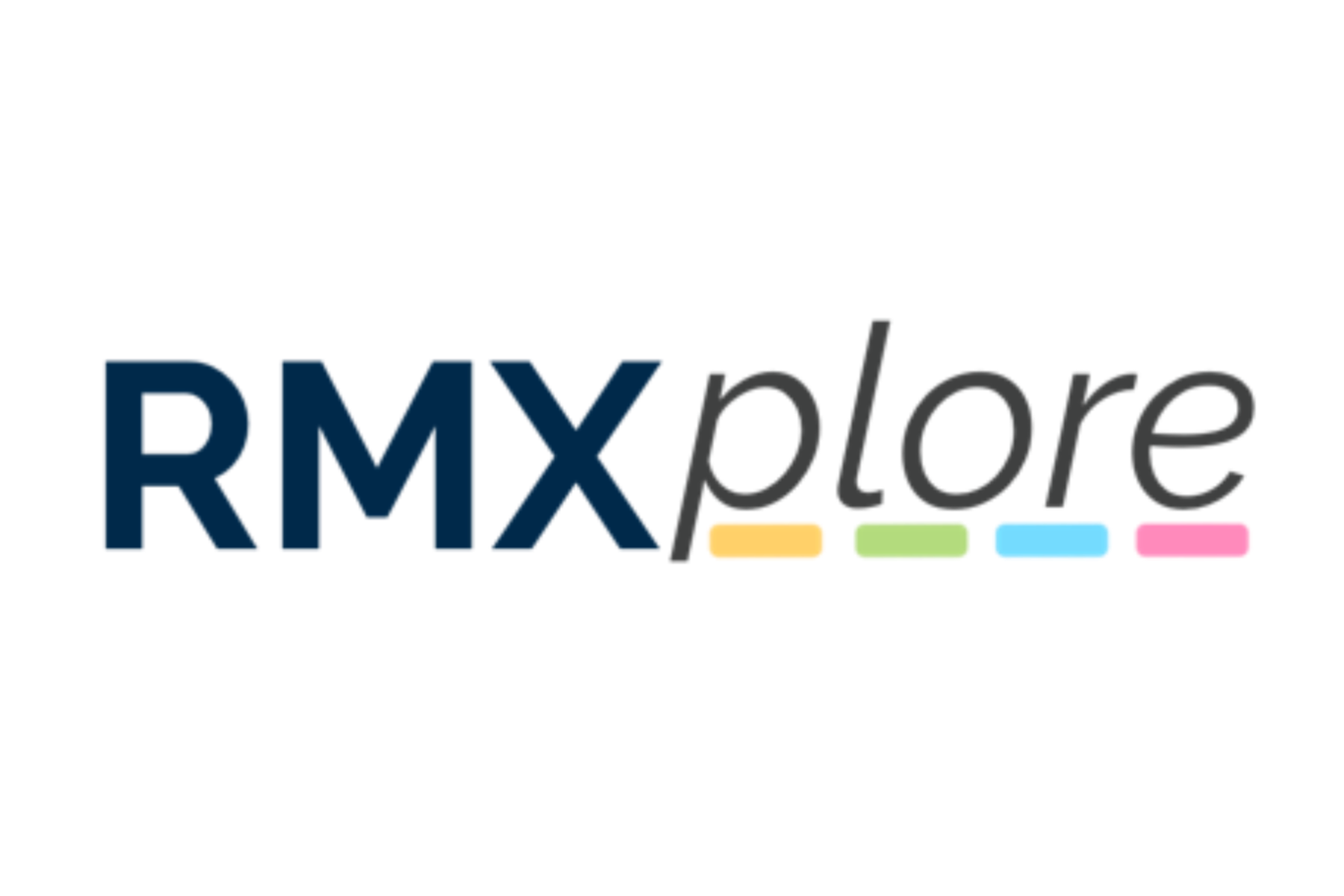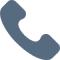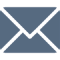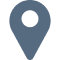## Operations, Measurement and Thinking (OMT) I

### Operations, Measurement and Thinking (OMT) I

Begins TBA
Course Pricing:
• Online : \$1540
• Cupertino Center : \$1540
Course Logistics:
Duration : 90 minutes
Number of Classes : 30 Classes
Occurs : Saturdays (9:00 - 10:30 a.m.)
<< Back To Courses

### Course Topics:

Course Topics are organized as six modules with five granular topics covered under each module. Click through below to see all modules/topics for this course.

### 01 - Additions and Subtraction• Number notation and place values
• Ordering numbers
• Introduction to Addition and subtraction (up to 20)
• Carry over
• Addition: 3 digit by 1 digit, 3 digit by 2 digit

### 02 - Multiplication and Division• Multiplication of 1-digit numbers
• Division into equal sets
• Repeated subtraction
• Multiplication and division up to 20

### 03 - Measurement• Measuring with a ruler
• Basic unit conversions
• Dealing with money: dollars to cents, etc.
• Telling and writing time at hourly and half-hour marks
• Introduction to length, mass, and volume

### 04 - Elementary Geometry• Basic shapes: triangle, quadrilateral, pentagon, etc.
• Rectangles and squares
• Circles: what is a radius?
• Recognize shape, size, and color
• Introduction to 3D Objects: cube, block, cylinder, cone

### 05 - Data Analysis• Organizing data
• Make picture graphs
• Use of symbol/picture to represent one object
• Interpret picture graphs
• Picture graphs with scales

### 06 - Word Problems